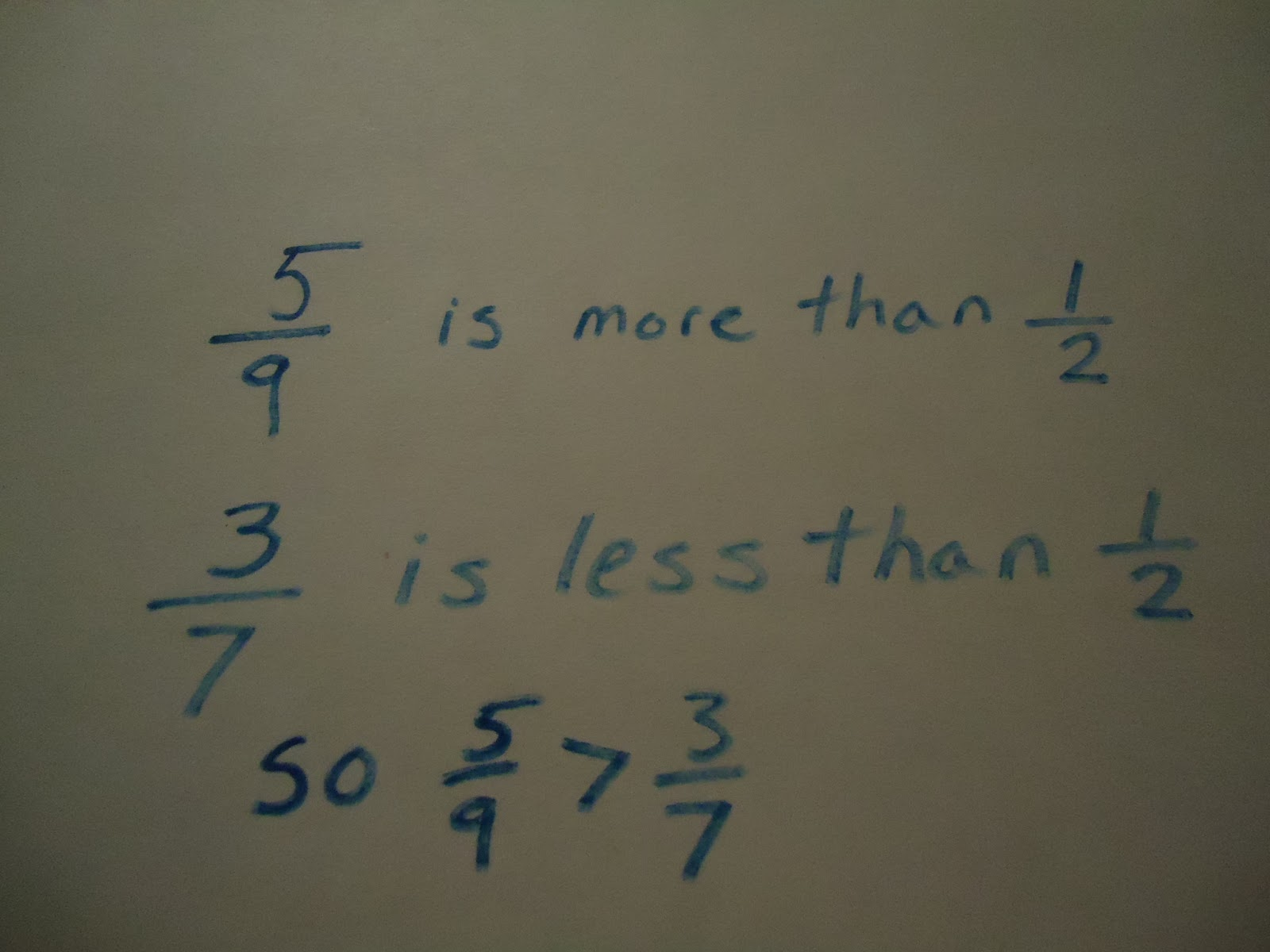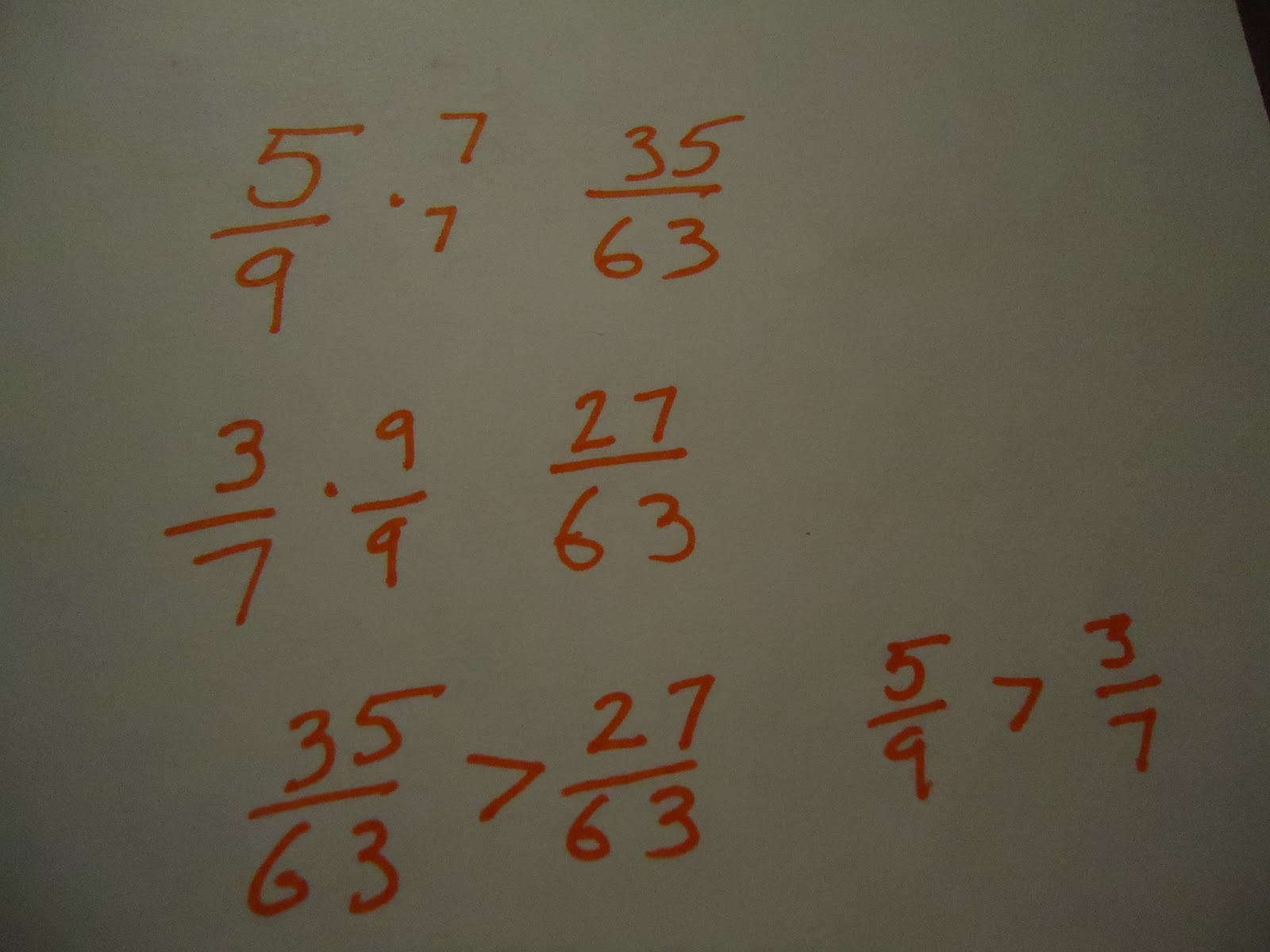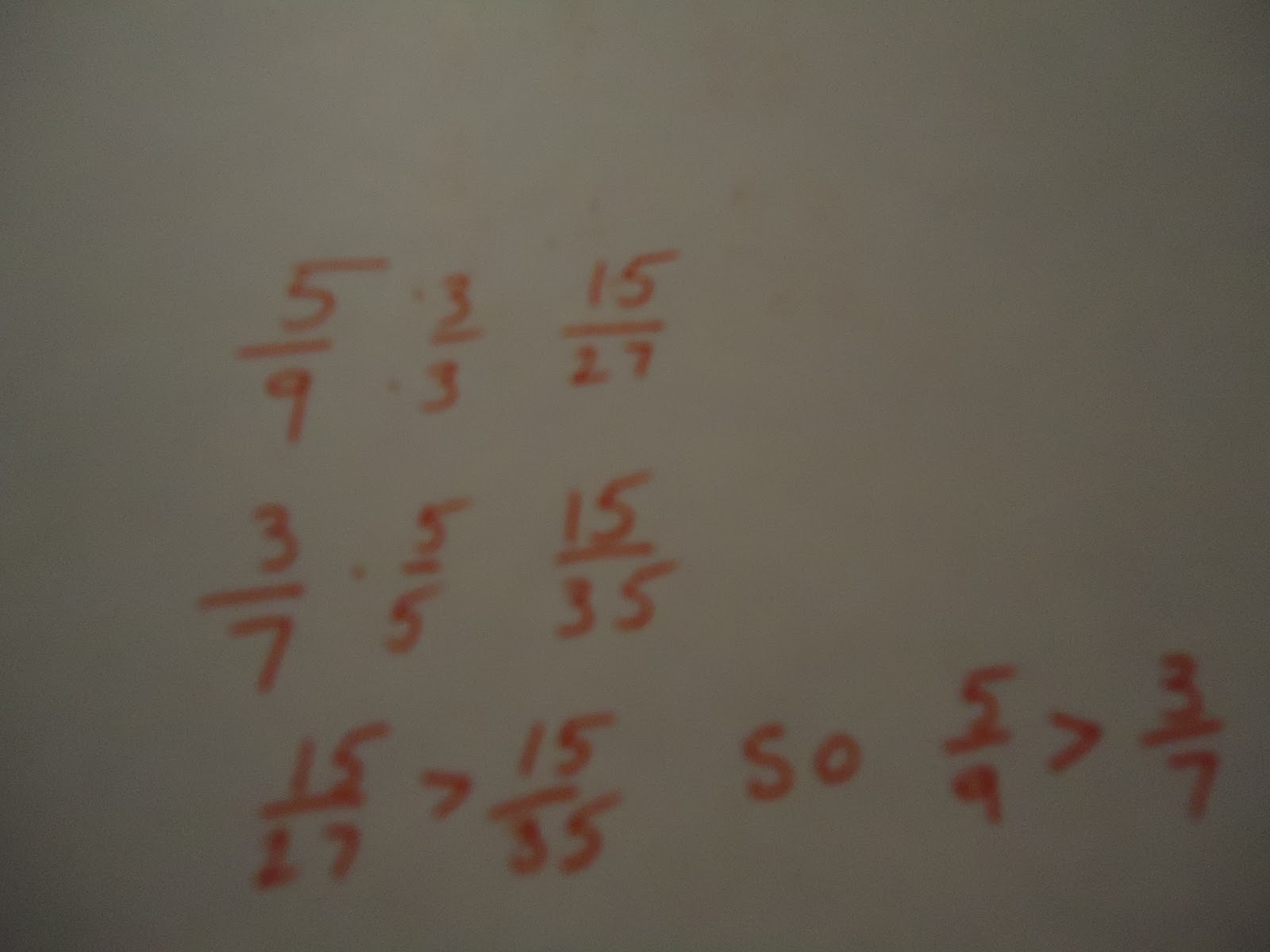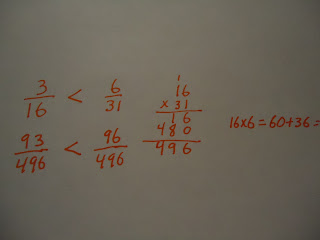## Tuesday, April 30, 2013

### Comparing Fractions with Fourth and Fifth Graders

One of the biggest changes for my school as we move toward the Common Core is that we have a lot more work to do with fractions in fourth and fifth grade.  I have been working with a mixed group of fourth and fifth graders on comparing fractions recently.  Here is what the common core says in regards to comparing fractions at grade 4:

CCSS.Math.Content.4.NF.A.2 Compare two fractions with different numerators and different denominators, e.g., by creating common denominators or numerators, or by comparing to a benchmark fraction such as 1/2. Recognize that comparisons are valid only when the two fractions refer to the same whole. Record the results of comparisons with symbols >, =, or <, and justify the conclusions, e.g., by using a visual fraction model.

I created this little fraction compare worksheet and chose fractions that I thought would elicit some of the different strategies suggested by the Common Core

Yes this is the entire thing!!!! I really wanted to focus on strategies used to compare these fractions.  Let's look at some of the strategies my students used for each one.  It is simple, but very effective!  I have made it available for free for anyone who wants to try it!  Click here to grab a copy!

#### The first one is 3/16 and 3/15

This questions is obviously written to illicit ideas about common numerators.  We spend so much time on common denominators that sometimes common numerators get completely ignored!

The majority of my students used unit fraction reasoning on this one.  They reasoned that we had 3 pieces for each one and since fifteenths are larger than sixteenths, 3/15 would be larger than 3/16.

A few of my students tried drawing a model for this.  Because these fractions are really close, it was hard to tell from their models which was larger because of the inherent margin of error with drawing models.  Model drawing is an important first step for kids who are still working on developing conceptual understandings of fractions.  It is not where I want to leave students at the end of grade 5, but it is an important first step!

#### Let's look at 5/9 and 3/7

The strategy I was trying to elicit with this one was comparing to the benchmark of 1/2.  Here are the four strategies used by my group to compare these fractionsHere was the strategy I was thinking of when I wrote the problem.  This student compared both fractions to the benchmark of 1/2.  Since one fraction is just  below 1/2 and the other just above it, this is a great strategy for this problem.This student drew a model.  As far as model drawing goes, this is fairly good.  This can not be the only strategy kids use to compare fractions but it is a great place to start and really helps build conceptual understanding of fractions.This student used a common denominator strategy to compare the fractions.  Common denominators will of course be something you want your fifth graders to be solid on, but please remember that it isn't the ONLY was to compare fractions and often times it is not the most efficient way.This student surprised me by using a common numerator strategy.  It is actually very efficient for this problem.  I didn't write the problem purposely to illicit this strategy but it works quite well.

####This student used reasoning about how far away from 1 these fractions are.  They used what they know about unit fractions to help them with this comparison.This student used a number line model to compare the fractions.  If you look closely, you will see the partitioning done in red was done to make sixths and the darker color was used to make eighths.  The number line is a model that I have found really effective for helping kids move forward with conceptual understanding around fractions.

#### The Last OneI chose this problem because it was really easy to create a common numerator for these numbers.  That is what this student did and it was very effective and efficient!This student used a common denominator strategy to compare these two fractions.  You can see that in this case it is much less efficient than finding a common numerator.

This took an entire class period but I got such great ideas and strategies out of the students.  I love days like this when kids have a lot of time to share strategies!

A GREAT resource if you want to really help your kids excel with fractions is this book A Focus on Fractions.  Reading it completely changed the way I thought about teaching fractions in grades 1-6.

What strategies do your students have for comparing fractions?

1.1.